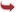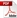International Journal of Scientific and Research Publications

#### IJSRP, Volume 2, Issue 9, September 2012 Edition [ISSN 2250-3153]EIENSTEIN FIELD EQUATIONS AND HEISENBERG’S PRINCIPLE OF UNCERTAINLY THE CONSUMMATION OF GTR AND UNCERTAINTY PRINCIPLE
DR K N PRASANNA KUMAR, PROF B S KIRANAGI , PROF C S BAGEWADI
Abstract: The Einstein field equations (EFE) or Einstein equations are a set of 10 equations in Albert Einstein general theory of relativity which describe the fundamental interaction (e&eb) of gravitation as a result of space time being curved by matter and energy. First published by Einstein in 1915 as a tensor equation, the EFE equate spacetime curvature (expressed by the Einstein tensor) with (=) the energy and momentum tensor within that spacetime (expressed by the stress–energy tensor).Both space time curvature tensor and energy and momentum tensor is classified in to various groups based on which objects they are attributed to. It is to be noted that the total amount of energy and mass in the Universe is zero. But as is said in different context, it is like the Bank Credits and Debits, with the individual debits and Credits being conserved, holistically, the conservation and preservation of Debits and Credits occur, and manifest in the form of General Ledger. Transformations of energy also take place individually in the same form and if all such transformations are classified and written as a Transfer Scroll, it should tally with the total, universalistic transformation.
[VIEW FULL PAPER][DOWNLOAD]

Reference this Research Paper (copy & paste below code):

DR K N PRASANNA KUMAR, PROF B S KIRANAGI , PROF C S BAGEWADI (2018); EIENSTEIN FIELD EQUATIONS AND HEISENBERG’S PRINCIPLE OF UNCERTAINLY THE CONSUMMATION OF GTR AND UNCERTAINTY PRINCIPLE; Int J Sci Res Publ 2(9) (ISSN: 2250-3153). http://www.ijsrp.org/research-paper-0912.php?rp=P09169